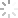服务体验• 服务承诺： 正品保障
• 公司名称：
• 所 在 地：
• 客服电话：超级新品 基础代数 9787510094644

• 作者： (英)卡恩(Cohn,P.M.)著 | 暂无编 | 暂无译 | 暂无绘
• 出版社： 世界图书出版公司
• 出版时间：2015-05-01

商品预定流程：

• 付定金
• >
• 商家备货
• >
• 付尾款
结束：6月30日23:59
• >
• 发货
预计7月1日发货

• 商品

• 服务

• 物流

为您推荐大家关注的

• 作者： (英)卡恩(Cohn,P.M.)著| 暂无编| 暂无译| 暂无绘
• 出版社：世界图书出版公司
• 出版时间：2015-05-01
• 版次：1
• 页数：465
• 开本：16开
• 装帧：平装
• ISBN：9787510094644
• 版权提供：世界图书出版公司

ISBN：9787510094644

《基础代数(英文)》由世界图书出版公司北京公司出版。

读者对象：数学专业的广大师生。

P.M.Cohn(P.M.卡恩,英国)是知名学者，在数学和物理学界享有盛誉。本书凝聚了作者多年科研和教学成果，适用于科研工作者、高校教师和研究生。

Condition （c） of Theorem 4.3.4 may also be expressed by saying that any exact sequence 0 → N → M → M／N → 0 splits； in other words， every short exact sequence with middle term M splits.This is equivalent to either of the following conditions on （4.2.2）：
（a）There is an R—homomorphism f'： M → M' such that ff' = 1M'； this mapping f' is called a right inverse or retraction for f.
（b）There is an R—homomorphism g' ： M' → M such that g'g = 1M'； the mapping g' is called a left inverse or a section for g.
Clearly （a）， （b） hold when im f is plemented； conversely， in case （a） we have M = im f □ ker f' and in case （b） M = ker g □ im g'， as is easily verified.
Over a field （even skew） the simple modules are just the one—dimensional vector spaces； since every vector space can be written as a sum of one—dimensional spaces， it follows that over a field every module （= vector space） is semisimple.In particular， this proves the existence of a basis for any vector space， even infinite—dimensional.For if V = □ISi and ui is a generator of S， then {ui}i∈I is a basis of V， as is easily checked.
For an arbitrary ring R the theory of semisimple modules is quite similar to the theory of vector spaces over a field.The main difference is that there may be more than one type of simple module.We shall say that two simple R—modules have the same type if they are isomorphic.A semisimple R—module is called isotypic if it can be written as a sum of simple modules all of the same type.In any R—module M the sum of all simple submodules is called the socle of M； thus the semisimple modules are those that coincide with their socle.The sum of all simple submodules of a given isomorphism type a is called the α—socle of M or a type ponent in M.
Let M be any R—module； a submodule N of M is said to be fully invariant in M if it admits （i.e.is mapped into itself by） all R—endomorphisms of M.We note that for an R—module M， the set S = EndR（M） is just the centralizer in End（M） of the image of R defining the R—action on M， so a subgroup N of M is a fully invariant submodule iff it admits both R and S.In a semisimple module the fully invariant submodules are easily described： they are the sums of type ponents.
Theorem 4.：1.7.Let R be a ring and M be an R—module.Then：
（ⅰ）For any type α the α—socle is an isotypic submodule of M containing all simple submodules of type α， and the socle is the direct sum of the α—socles， for different α.